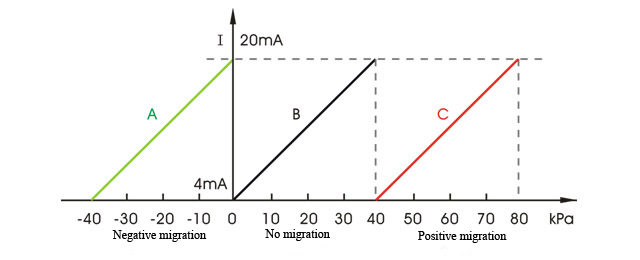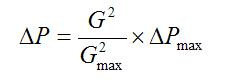Pressure Sensor

Home » Application » Improve Pressure Sensor Measurement Accuracy by Zero Migration

# Improve Pressure Sensor Measurement Accuracy by Zero Migration

In order to improve the measurement accuracy, pressure sensors mostly have a zero migration device, which can migrate the zero point of the sensor, that is, the starting point of the range, in the positive and negative directions. In practical applications, migration can be divided into three types: no migration, negative migration, and positive migration.
The measuring range of the sensor is equal to the sum of the range and the migration amount, that is, the measuring range = range + migration amount. As shown in the figure, the A range is 40 kPa, the migration amount is -40 kPa, and the measuring range is -40 to 0 kPa; B range is 40kPa, no migration, the measuring range is 0-40kPa; The C range is 40 kPa, the migration amount is 40 kPa, and the measuring range is 40-80 kPa.As can be seen from the figure, the input and output characteristic curves of positive and negative migrations are characteristic curves without migration amount and pan along the abscissa that indicates input. Therefore, the essence of positive and negative migration is to adjust the upper and lower limits of the range by calibrating the sensor, and the range is unchanged. It can also be understood that when the input pressure is zero (0%), the output current signal is also zero (0%), which is no migration. When the input pressure is zero (0%), the output current signal is positive, greater than the working zero, which is negative migration. When the input pressure is zero (0%), the output current signal is negative and less than the working zero, which is a positive migration.
Not all sensors have migration capabilities, such as conventional temperature sensors with little migration. Using the migration most widely are pressure sensors and differential pressure sensors, which are determined by the need for use and the primary part structure. However, it is difficult to achieve migration for a differential pressure sensor with square function.
So, when it is necessary to perform a zero-point migration for the pressure sensor?

1. When measuring the liquid level in the closed container

When using the pressure sensor to measure the liquid level in the closed container, if the gas phase medium is easy to condense, the condensate entering the pressure guiding tube and the negative pressure chamber in the sensor will cause the liquid index in the negative pressure chamber change, thereby causing measurement error. Therefore, the solution is generally to install a balance vessel in front of the conduit above the negative pressure chamber and fill the balance vessel with condensate. When measuring harmful or corrosive medium, in order to prevent the medium from entering the sensor, it is also necessary to install the isolator and fill the pressure guiding tube with the isolation fluid. In both of the above measurements, the presence of condensate will cause the signal of the negative pressure chamber of the sensor to be greater than the positive pressure chamber signal, resulting in failure to measurement. The method that  reverse the positive and negative pressure ports of the sensor can be used, but the sensor output is decreased when the liquid level rises, which easily causes an illusion and is inconvenient to connect with the control system.
To solve this problem, it is necessary to use a negative migration to start the zero point of the sensor from the negative differential pressure, so that it can meet the use requirements.

2. Pressure sensor installation position is lower than the pressure point

Generally, the pressure sensor are installed below the pressure tapping point, and the pressure guiding tube is completely filled with condensed water. Due to the water column static pressure, the sensor output current contains the water column static pressure. The greater the vertical distance between the pressure point and the pressure sensor, the greater the error.
When measuring the liquid level in the open container, if the pressure sensor is installed under the container, the measurement error will also occur due to the influence of the liquid column static pressure of the measured medium, and the result is the same as above.
To solve the above problem, it’s necessary to use positive migration: Adjust the pressure sensor output to zero (4 mA), which is equivalent to subtracting the liquid column static pressure. The pressure sensor output value after the positive migration is the actual working pressure value.

3. Compress the range by migration to improve measurement accuracy

For example, there is a 35t/h industrial boiler, and its gas consumption is always above 25t/h. In order to improve the flow measurement accuracy, we can change the test range of the display instrument to 20-40t/h, and the pressure sensor compress its range by the positive migration to match it. It is known that when G=40t/h, ΔP=60kPa, it can be calculated by the following formula:In the formula, ΔPmax is the upper limit of the differential pressure of the meter; ΔP is any differential pressure; G is the flow of any mass; and Gmax is the mass flow of the meter at the upper limit of the differential pressure.
According to the above formula, when the flow G=20t/h, the corresponding differential pressure △P=15kPa; when the flow G=40t/h, the corresponding differential pressure △P=60kPa. Then the range of the pressure sensor is reduced to 0-45kPa. Positive migration is performed to reduce the measurement range of the sensor to 15-60 kPa, and the corresponding flow is 20-40 t/h, which improves the measurement accuracy.
As can be seen from the above examples, the measurement requirements vary depending on the measurement specification and equipment. This requires the pressure sensor to have a migration function, and it is convenient for the user to migrate on the spot according to the actual situation to adapt to various measurement requirements in the production.

I have an Opinion:

Plz Calculate (2 + 8) =
(Your comment will show after approved.)

You may also like: